Courses
Courses for Kids
Free study material
Free LIVE classes
More

# Counting in 2s Missing Numbers Number LineLIVE
Join Vedantu’s FREE Mastercalss

## Introduction to Counting in 2s

Learning to add numbers by 2 is done through skip counting by twos. We begin by skip counting and adding when we first gain the concept of addition. Skip counting by 2 is adding 2 to each previous number to obtain the next number in the series. This method helps us in learning the concept of the addition of 2. Starting with 0, skipping counting by two results in a series that looks like \$0, 2, 4, 6, 8\$ and so on.

Skip counting is a mathematical strategy taught as a sort of multiplication. Earlier textbooks referred to this method as "counting by twos" (threes, fours, etc.). A person can count to ten using only the other even numbers \$2,4,6,8\$ and 10 when skip counting by 2s.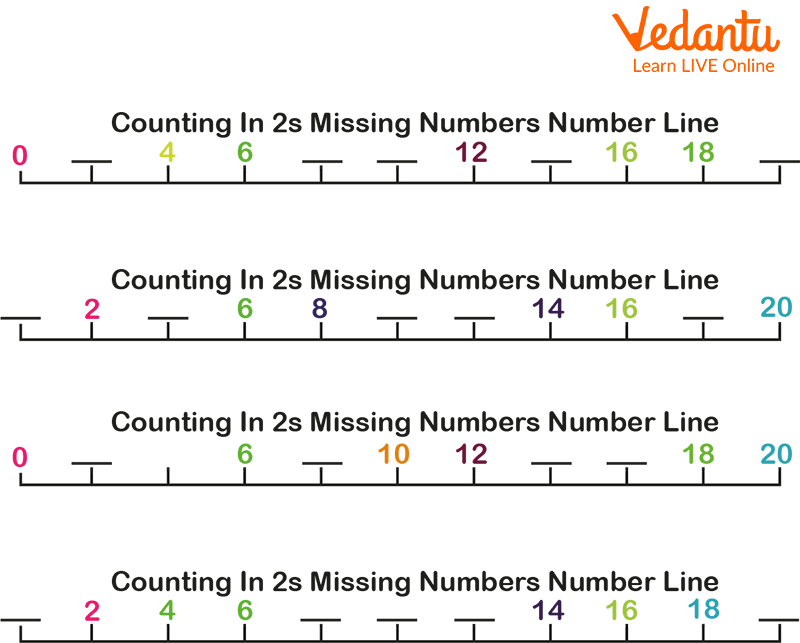# Counting in 2s

## Counting in 2s

To skip count by two, add two to each previous number to get the subsequent number in the sequence. This skill helps in our understanding of the addition of two concepts. When learning addition by counting, skip counting by two is a highly helpful skill that advances our understanding of addition by adding two to each number. The table of two is easier to memorise when we skip count by two.

## Skip Counting by 2 on Number Line

In this section, we will learn the concept of skip counting by 2 on a number line. We will make two jumps to reach one number to another. Let us have a look at the number line below. Starting from the number 0, when skip counting by 2, the next number will be 0 + 2 = 2.

So, we have landed on the number 2. Next, when we take another jump, we land on 2 + 2 = 4. Continuing in the same manner, we skip a number and land on the next number. So, the series continues like 0, 2, 4, 6, 8, 10, and so on.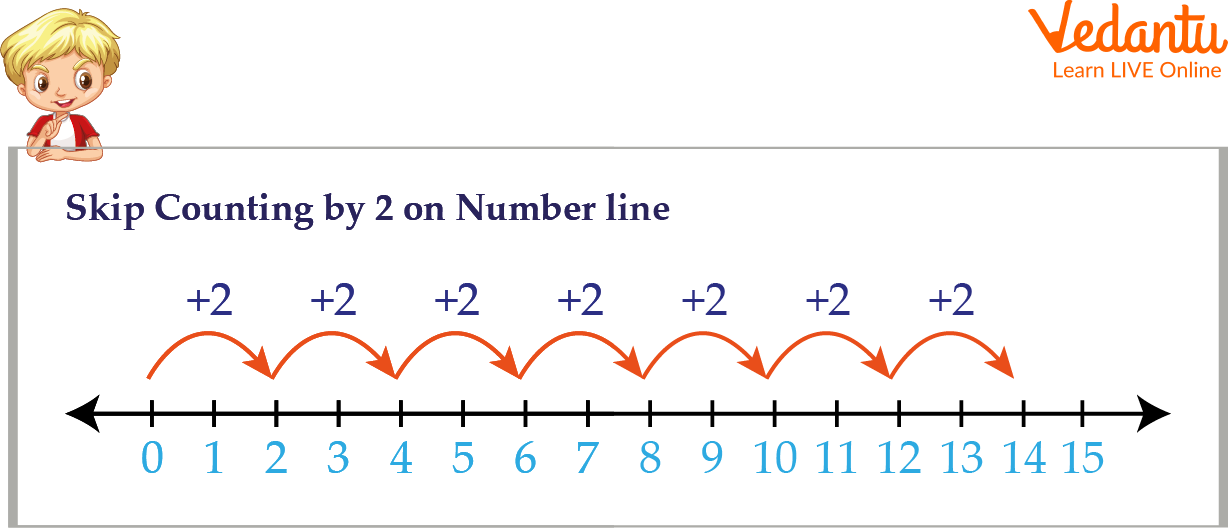Skip Counting by 2 on Number Line

Find the missing numbers in the series 12, ___ , ___, 18, 20, 22, ___ using skip counting by 2.

Ans: To find the missing numbers by skip counting by 2, we add to each number in the series to obtain the next number.

12 + 2 = 14

14 + 2 = 16

22 + 2 = 24

So, the series is 12, 14, 16, 18, 20, 22, 24

The missing numbers are 14, 16 and 24.

Example: Write the multiples of 2 up to 20.

Ans: We will use the method of skip counting by 2 to write the table of 2. The first multiple is 2, so we obtain the remaining multiples by adding 2 to each multiple.

2 + 2 = 4

4 + 2 = 6

6 + 2 = 8

8 + 2 = 10

10 + 2 = 12

12 + 2 = 14

14 + 2 = 16

16 + 2 = 18

18 + 2 = 20

So, the multiples of 2 up to 20 are 2, 4, 6, 8, 10, 12, 14, 16, 18, 20.

## Counting in 2s Activities

###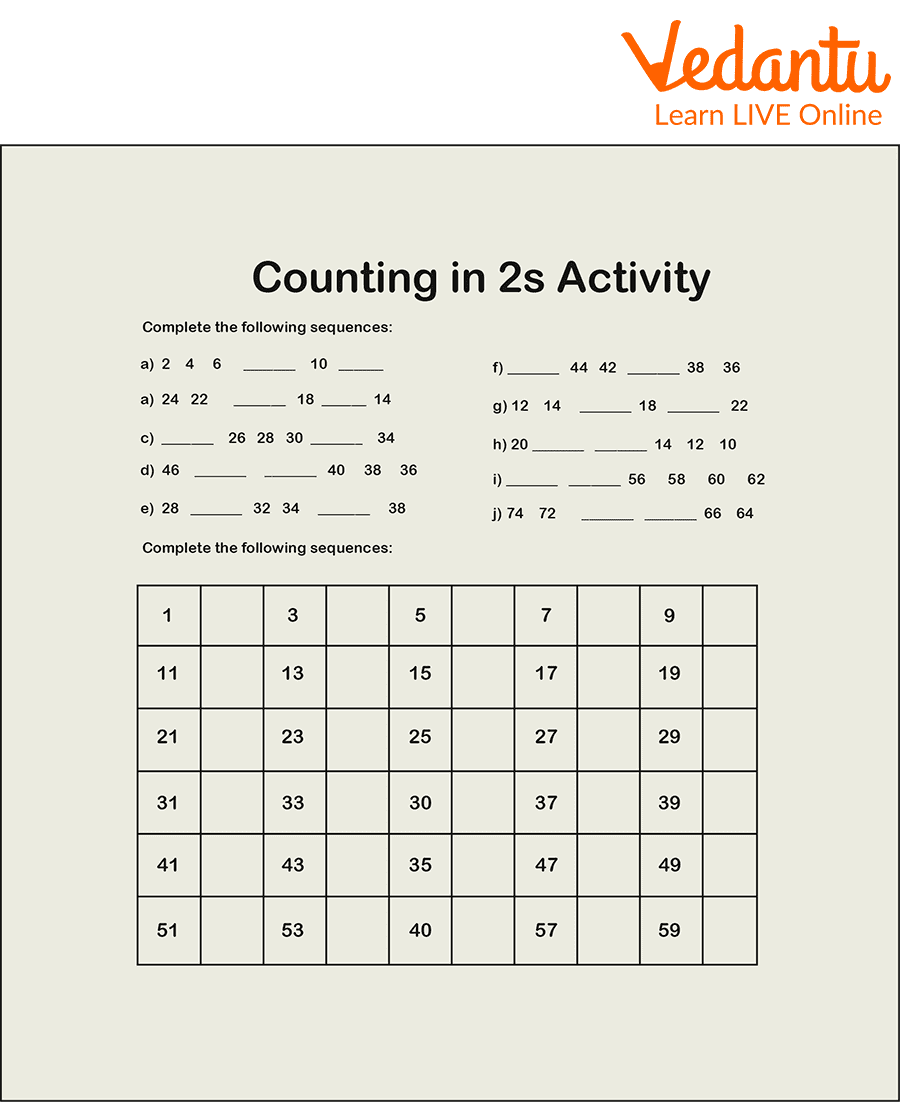Counting in 2s activities

## Counting in 2s Game

Write all the multiples of two which are not circled.

###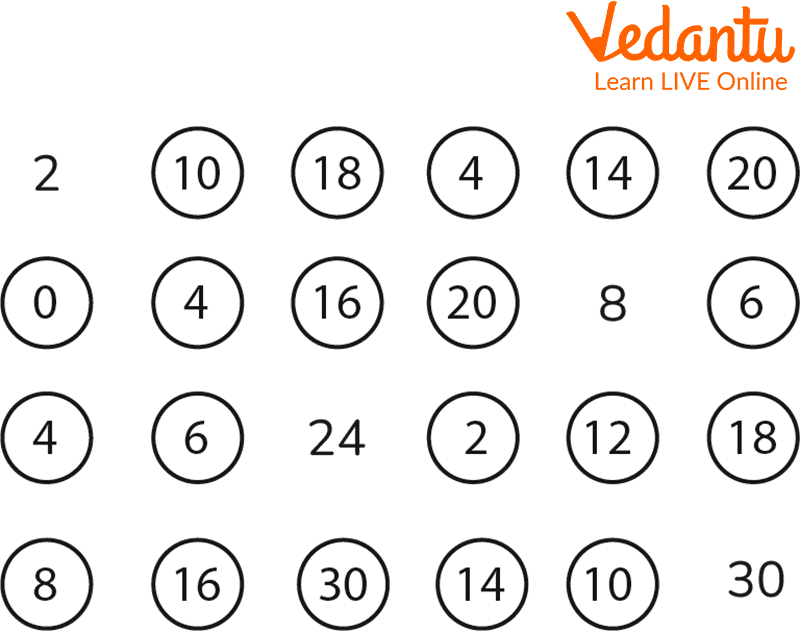Counting in 2s game

Ans: Multiple of 2s, which are not circled, are as follows:

2, 8, 24 and 30.

## Counting in 2s Worksheet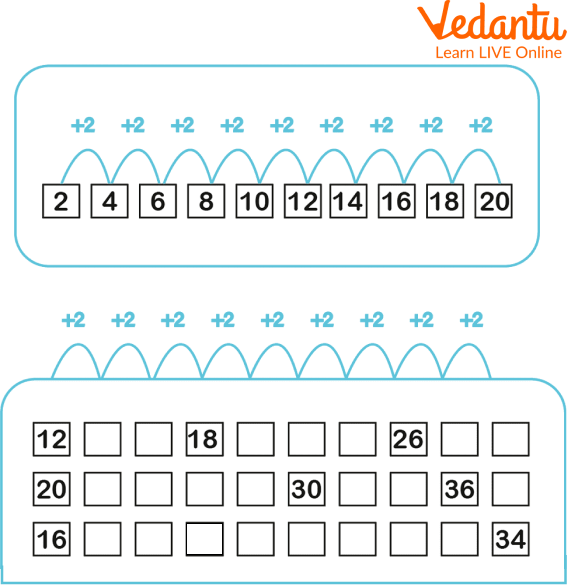Counting in 2s worksheet

Ans:## Summary

To conclude all the conceptual understanding regarding Counting in 2s in this article, we can say the idea of skipping two numbers on a number line. To get from one number to another, we have to make the jump of two. Then, later on, we saw some examples based on skip counting, like the missing number between the multiple of 2s. Also, to get more clarity on the topic, we have included the activity worksheet and games through which students will get more engaged with counting in 2s.

Last updated date: 28th Sep 2023
Total views: 78.3k
Views today: 0.78k

## FAQs on Counting in 2s Missing Numbers Number Line

1. Why is it necessary to count in 2s?

Children's ability to recognise patterns and solve problems is boosted by doing this. It helps in learning the tables. They can better comprehend the idea of even and odd when they count in twos.

2. Kids should count by 2s when?

Your child's developing mathematical knowledge heavily relies on numbers and counting. These fundamental mathematical ideas lay the groundwork for later, more sophisticated mathematical operations. Eight-year-olds frequently count up to 1,000 and have mastered the art of skip counting (counting by 2s, 5s, 10s).

3. When should children start counting by 2s?

A youngster can first recognise when there is one and more than one, but not whether there are two or six. By the time a youngster is two years old, he can count to two ("one, two"), and by the age of three, he can count to three. However, if he can count to ten, he is probably reciting from memory.